# Graph Plotter :: An Online Graphing Calculator

### Ideas for activities

Can you draw pictures with graphs?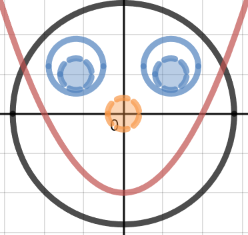Model real life situations by translating them into algebraic equations and by using graphs.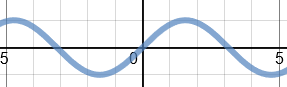Find approximate solutions of simultaneous linear equations using graphs. For example

y = 5x - 7

3x + 2y = 1

Plot families of exponential and reciprocal graphs. For example

y = 2x, y = 3x, y = 4x ...

y = 1÷x, y = 2÷x, y = 3÷x, ...

Reduce a given linear equation in two variables to the standard form y = mx + c; calculate gradients and intercepts of the graphs and then plot them to check.

Solve problems involving direct and inverse proportion using graphical representations.Identify and interpret roots, intercepts and turning points of quadratic functions graphically.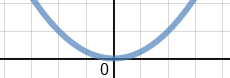Find the equations of the translations and reflections of graphs of given functions.Use graphs to solve optimisation problems.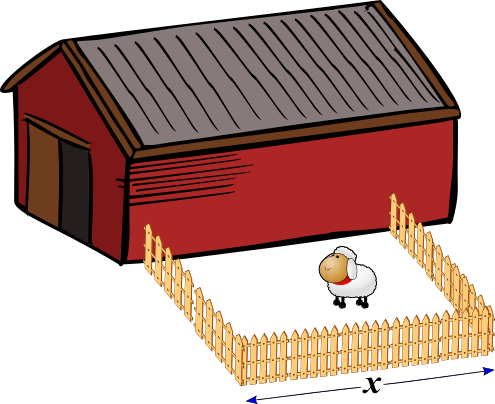Investigate the absolute value function. Plot families of graphs and describe their characteristics. For example

y = |x2 - 5|

Graph inequalities to solve linear programming problems. more...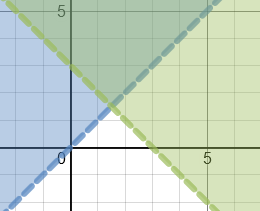Plot the graphs of functions and their inverses by interchanging the roles of x and y. Find the relationship between the graph of a function and its inverse. Which functions inverses are also functions?

### Related activities

#### Straight Line Graphs10 straight line graph challenges for use with computer graph plotting software or a graphical display calculator.

Transum.org/go/?to=straight

#### Graph MatchMatch the equations with the images of the corresponding graphs. Linear, quadratic and more.

Transum.org/go/?to=graphmatch

#### Human GraphsStudents should be encouraged to stand up and make the shapes of the graphs with their arms.

Transum.org/go/?to=humangraphs

#### Plotting Graphs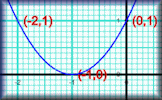Complete a table of values then plot the corresponding points to create a linear, quadratic or reciprocal graph.

Transum.org/go/?to=plotting

#### CoordinatesThis is a good place to start if you are new to this topic. Learn to read and plot coordinates.

Transum.org/go/?to=coordinates

#### Coordinates PictureYou will need plenty of practice plotting coordinates and these pictures make practice fun.

Transum.org/go/?to=cpc

#### Graph Equation Pairs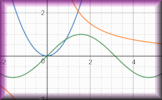Match the equation with its graph. Includes quadratics, cubics, reciprocals, exponential and the sine function.

Transum.org/go/?to=greqpairs

#### Graph SnapsComplete the tables and find the equations of the graphs that can be seen in the snaps.

Transum.org/go/?to=donsgraphsnaps

The Batman Equation - Turn Anything into a Graph - Sen Zen

Tuesday, October 24, 2017

Tom Lucas, Bristol

Wednesday, February 21, 2018

"It would be nice to be able to draw lines between the table points in the Graph Plotter rather than just the points."

Emmitt, Wesley College

Monday, July 22, 2019

"Would be great if we could adjust the graph via grabbing it and placing it where we want too. thus adjusting the coordinates and the equation."

Thursday, September 19, 2019

"Thank you very much for this program. It is excellent."

João Ricardo Oliveira, Brazil

Wednesday, April 22, 2020

"First of all, congratulations for the beautiful job! But I have a question: is there a way to save/download the graphics?

[Transum: Try doing a screen grab or, if you use Windows, use the Snipping Tool]"

Alistair, UK

Monday, October 12, 2020

"Absoultely brilliant got my kids really engaged with graphs. Unfortunately it only plots the positive answer to the square root so the circles would not plot.

[Transum: Glad to hear they were so engaged. Yes the positive square root is the default. Try plotting the circle with the equation in the form x2 + y2 = 52 see here]"

Soumi Dana,

Sunday, January 31, 2021

"Hello, I am Soumi Dana, currently studying in 8th grade. I am very thankful for Transum and through this, my passion for math has increased. Although I don't understand much about trigonometric graphs, I have plotted some trigonometric graphs with beautiful patterns. When I know more about Trigonometry I will understand why these graphs are the way they look. For now, I love plotting them even though I don't understand them well.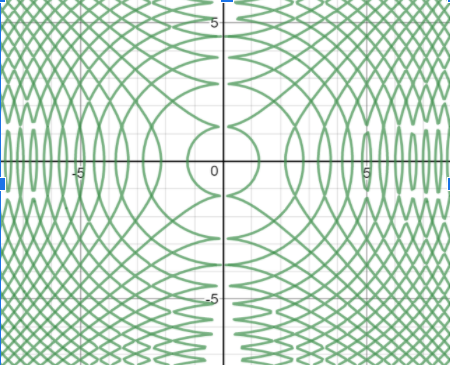The graph above is an example. When writing the equation, cos(x²)=sin(y²) the following graph is plotted. I find it mesmerizing that an equation can give amazing results. Thank you for making Transum free and available on the internet. I am very grateful for this site. Thank you, Soumi."

Akshat, India

Saturday, March 6, 2021

"Can't we plot parametric equations in this plotter?

[Transum: Yes, enter them as coordinates with the variable t. This example will draw a circle: (sin t, cos t).]"

Paul DeQuattro, Pauldeq@gmail.com, Los Angeles

Monday, May 17, 2021

"My question may not seem mathematical in nature, but here goes: mechanics and auto technicians are stumped trying to find the problem with my car engine that results in the check engine light being ON all the time. I've assumed my problem is not typical and I'm searching for a clue, any clue. I've discovered there may be some sort of linear relationship between two time periods (P1 and P2), and I'd like to show it on a graph and I'm seeking help in that regard. P1 is the time period in days or partial days wherein the car battery is completely disconnected; P2 is the time period, upon reconnecting the battery, wherein the check engine light remains OFF until it illuminates ON. My experiments have shown where P1 is greater, P2 is greater. Where P1 is a few minutes, P2 is a few minutes; where P1 is overnight (20 hours), P2 is 2-3 days; P1 is 5 days, P2 is 11 days; P1 is 10 days, P2 is 22 days; and finally, P1 is 5+ months, P2 is 5+ months. When people hear my story, their eyes glaze over and they turn off listening. I'd like to show a graph to an expert (such as an automotive electrical circuit engineer, if there is such a thing) and ask him/her to explain the relationship in my experiments. Thanks if you can comment on graphing this. Thanks for reading even if you can't"

Do you have any comments? It is always useful to receive feedback and helps make this free resource even more useful for those learning Mathematics anywhere in the world. Click here to enter your comments.For All: